### Home > CALC > Chapter 5 > Lesson 5.3.2 > Problem5-124

5-124.
1. Suppose you deposit \$50 in Piggy Bank, which pays 5.5% annual interest. How much money will be in your account: Homework Help ✎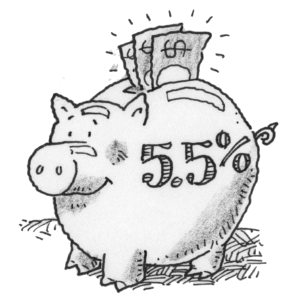1. After 6 months if the interest is compounded twice a year?

2. After 1 year if the interest is compounded twice a year?

3. After 1 year if the interest is compounded monthly?

4. After 2 years if the interest is compounded monthly?

5. After 2 years if the interest is compounded daily?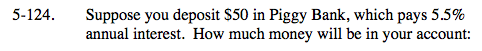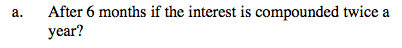$50\left(1+\frac{0.055}{2}\right)^1=\underline{\ \ \ \ \ \ }$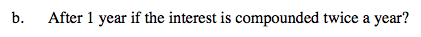Refer to hint in part (a).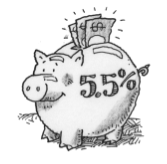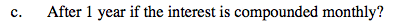$\text{investment }\left(1+\frac{\text{rate}}{\text{times compounded}}\right)^{12}=\underline{\ \ \ \ \ \ }$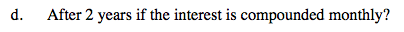Refer to hint in part (c).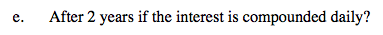Refer to hint in part (c).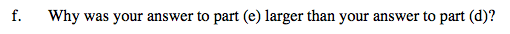Which Piggy Bank plan would you choose?